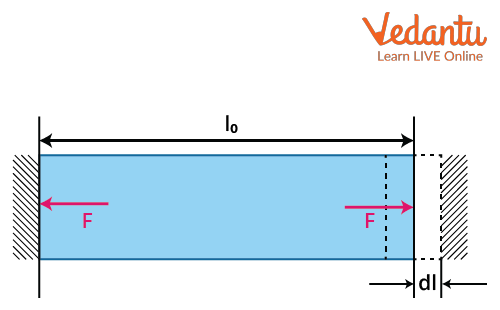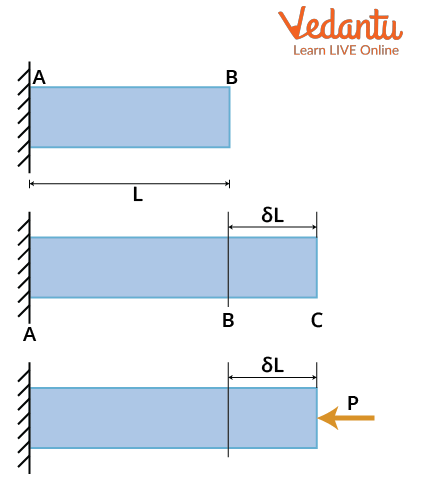Courses
Courses for Kids
Free study material
Free LIVE classes
More

# Thermal Stress and StrainLIVE
Join Vedantu’s FREE Mastercalss

## An Introduction to Thermal Stress and Strain

Thermal stress and strain are the properties of a material that are witnessed with an increase or decrease in temperature. Thermal stress is developed when the material is not allowed to expand or contract freely with the increase or decrease in temperature. The corresponding developed in this case is called thermal strain. Stress and strain of a material are both correlated with one another using the Young’s Modulus.

## Thermal Stress

Let us first understand in depth what thermal stress is. As mentioned earlier, thermal stress is the property of a material when its dimensions change with the increase or decrease in temperature, yet it is not allowed to expand or contract freely.Thermal Stress

As shown in the figure when the heat is applied or as there is an increase in the heat, the length of the rod expands by dl as seen in the figure above and the reverse will happen when there will be a decrease in the heat.

## Thermal Strain

Thermal strain is a property of material wherein a material is allowed to contract or expand freely with the increase or decrease in temperature. Thermal stress causes the body to produce thermal strain.

### Thermal Stress Formula

Consider a thermal conducting rod that expands when heated. The length change will be proportional to the amount of heat provided and the coefficient of thermal expansion. As a result, we can express thermal stress mathematically as:

\begin{align} &\delta_{T}=L \Delta T \\ &\delta_{T}=L\left(T_{f}-T_{i}\right) \end{align}

where

L denotes the length in metres.

Ti is the initial temperature in degrees Celsius.

Tf is the final temperature in degrees Celsius.

T=Tf-Ti is the temperature change in degrees Celsius.

α is the coefficient of thermal expansion.

The rate at which an object's shape/physical dimensions change in response to temperature changes is defined as the coefficient of thermal expansion. It varies depending on the material. It measures the fractional change in length of material per degree rise in temperature, keeping the pressure constant.

According to the laws of thermodynamics, thermal stress is a property caused by the internal temperature or the bar. However, under normal conditions, if there is a change in the external temperature, it further causes thermal stress. If this process continues, it leads to thermal shock.

## Thermal Stress and Strain Derivation

Consider the metal bar AB depicted in the figure below. Assume that the metal bar's initial temperature is T1 and that we are heating the metal bar at one end to achieve the final temperature of the metal bar, T2. Let us suppose the metal bar's initial length is L.Thermal stress in a rod

As previously stated, heating the material will result in an increase in temperature and, as a result, an increase in the dimensions of the material.

Increase in temperature of the metal bar, $\Delta$T = T2-T1

Assume that the material's free expansion due to temperature rise is not restricted, i.e., free expansion of the material is allowed, as shown in figure BC. Also, Assume that the free expansion caused by the increase in temperature of the metal bar due to heating is $\delta$L. The following formula, as shown here, will provide free expansion.

$\delta L=\alpha . L . \Delta T$

Thermal strain will be determined using the following result, as written here, with the help of the following result.

Thermal strain, $\varepsilon=\dfrac{\delta \mathrm{L} }{ \mathrm{L}}$

Thermal strain, $\varepsilon = \alpha. \Delta T$

## What is Young's Modulus?

Young's Modulus of elasticity, denoted as E, measures the stiffness of a material or a body, when it is subjected to a force to alter its dimensions lengthwise.

Or

Young's Modulus of a material or an object is defined as its tendency, as to how quickly it can stretch and deform. It is defined as the ratio of tensile stress to tensile strain.

The numeric value of Young's Modulus denotes either high stress or low strain. Thus, its value is fundamental. For example, rubber can easily be deformed and lower stiffness. Thus, the Young’s Modulus value of rubber is low as compared to steel or diamond

## Solved Examples on Thermal Stress and Strain

Example 1: A uniform cylindrical rod of length L and radius r is made from a material whose Young’s modulus of Elasticity equals Y. When this rod is heated by temperature T and simultaneously subjected to a net longitudinal compressional force F, its length remains unchanged. The coefficient of volume expansion of the material of the rod is nearly equal to?

Solution:

$Y = \left(\dfrac{F}{\pi r^2}\right) \times \dfrac{L}{\Delta L}$

$\Delta L = \dfrac{Fl}{\pi r^2Y}$         …….(1)

Change in length due to temperature change is given by

$\Delta \mathrm{L}=\mathrm{L} \alpha \Delta \mathrm{T}$               …….(2)

From equation (1) and (2),

$L \alpha \Delta T = \dfrac{FL}{AY}$

$\alpha= \dfrac{F}{AY\Delta T}$

$\alpha = \dfrac{F}{\pi r^2YT}$

Coefficient of volume expansion

$3\alpha = \dfrac{3F}{\pi r^2YT}$

Hence, the coefficient of volume expansion of the material of the rod is nearly equal to $\dfrac{3F}{\pi r^2YT}$.

Example 2:  What will be the pressure that has to be applied to the ends of a steel wire of length 5 cm to keep its length constant when its temperature is raised by 50 K? (For steel Young’s modulus is 2$\times$1011 Nm-2 and coefficient of thermal expansion is 1.1$\times$10-5 K-1)

Solution:

Given, $\Delta$ T = 50 K, Y =2$\times$1011 Nm-2

$\alpha=1.1 \times 10^{-5} K^{-1}$

Thermal strain in the wire $= \alpha \Delta T$

(As we have  $l = l_0 (1 +\alpha \Delta T)$)

Thermal stress in a rod is the pressure due to the thermal strain.

Required pressure $=Y \alpha \Delta T=2 \times 10^{11} \mathrm{Nm}^{-2} \times 1.1 \times 10^{-5} \mathrm{~K}^{-1} \times 50 \mathrm{~K}$

That gives $P =1.1\times10^8~Pa$

Hence, the value of pressure that has to be applied to the ends of a steel wire is $1.1\times10^8~Pa$.

## Conclusion

It can be seen from above that thermal stress and strain are fundamental properties of a material. Both are dependent on temperature. The rise or fall in temperature changes its dimensions. A major difference between the two is that the material in one is allowed to expand or contract freely, whereas the same is not the case for the other. Thermal stresses lead to deformation in the material, which further depends on material types and constraints. Stress induced in a material gives rise to strain. A detailed overview of how the temperature changes the dimensions and the relationship between stress and strain is discussed above.

Last updated date: 30th Sep 2023
Total views: 3.3k
Views today: 0.03k

## FAQs on Thermal Stress and Strain

1. What are the different types of thermal stresses?

Thermal stresses can be further divided into tensile and compressive stress, hydraulic and tangential or shear stress. The types of stresses depend upon the action of force that is deforming the material and how the orientation of the body changes on the application of the applied force.

Tensile strength of a material is when two equal and opposite forces are applied on a body to increase its length, the stress is termed as tensile stress, whereas when two equal and opposite forces are applied on a body to decrease its length, it is termed as compressive stress.  The thermal strain on the other hand can be divided into longitudinal strain, shear strain, and volume strain, respectively.

2. What is thermal shock?

Thermal shock is somewhat related to thermal stress. It is the sudden change in temperature, which causes a change in the tension in the material. The change in temperature causes tension in the material, which could lead to its deformation or a fracture in the material. Thermal shock and the changes produced by it take place suddenly. The temperature suddenly could change from hot to cold or vice versa. Thermal shock suddenly breaks the material and is especially common in brittle materials like ceramics.